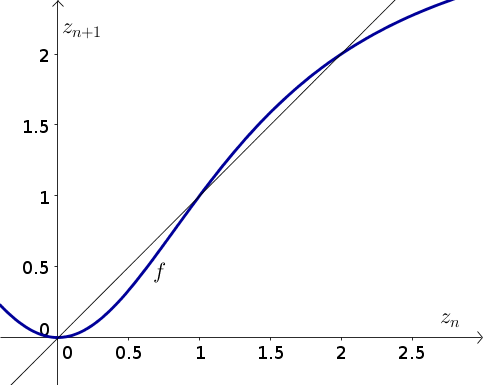# Math Insight

### Image: Discrete dynamical system example function 3The function $z_{n+1}=f(z_n)=\frac{3z_n^2}{2+z_n^2}$ is plotted in blue along with the diagonal line $z_{n+1}=z_n$.

Source image file: discrete_dynamical_system_example_function_3.ggb
Source image type: Geogebra